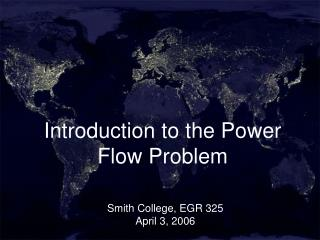Download PresentationIntroduction to the Power Flow Problem

# Introduction to the Power Flow Problem - PowerPoint PPT Presentation

Introduction to the Power Flow Problem. Smith College, EGR 325 April 3, 2006. Objectives. Introduce the power flow problem Numerical solution – issues? Investigate two power flow programs PowerWorld MATPower. V. I. Complex Power – Phasor Domain. IMPORTANT  is the power factor angle.I am the owner, or an agent authorized to act on behalf of the owner, of the copyrighted work described.
Download Presentation## Introduction to the Power Flow Problem

Download Policy: Content on the Website is provided to you AS IS for your information and personal use and may not be sold / licensed / shared on other websites without getting consent from its author.While downloading, if for some reason you are not able to download a presentation, the publisher may have deleted the file from their server.

- - - - - - - - - - - - - - - - - - - - - - - - - - E N D - - - - - - - - - - - - - - - - - - - - - - - - - -
Presentation Transcript
1. Introduction to the Power Flow Problem Smith College, EGR 325April 3, 2006

2. Objectives • Introduce the power flow problem • Numerical solution – issues? • Investigate two power flow programs • PowerWorld • MATPower

3. V I Complex Power – Phasor Domain IMPORTANT is the power factor angle Real Power Reactive Power

4. Power Flow Analysis • We know • The system topology (the circuit diagram) • The impedance of each line • The load at each load bus (S = P + jQ) • The capability of each generator (P, V) • We want to know • The output of each generator (S) • The voltage at each bus (V = V) • The power flow on each line (Pflow)

5. Real Power Flow Equations

6. Power Flow Analysis • Data needs? • Uses?

7. Real Power Flow Equations • How many equations and how many unknowns? • Numerical methods • Lack of convergence • Slack bus • Definition • Mathematical and physical role

8. Running a Power Flow Program • Programs created in the days of FORTRAN programming • Input data one of two standard formats • IEEE • PTI • Output data format designed by the programmer • ** Cases may or may not converge! **

9. Running a Power Flow Program • We will use two popular programs • MATPower • tabular data input and output • relatively easy to use • PowerWorld • visual • more difficult to use • Terminology • One-line diagrams • per unit system (normalize all values)

10. Power System Diagrams • Circuit vs. one-line diagram Arrows are used to show loads Generators are shown as circles Transmission lines are shown as a single line

11. Power System Diagrams • Circuit vs. one-line diagram

12. Summary • Introduction to the power flow problem and programs • Numerical methods • Significance of lack of convergence • Slack bus